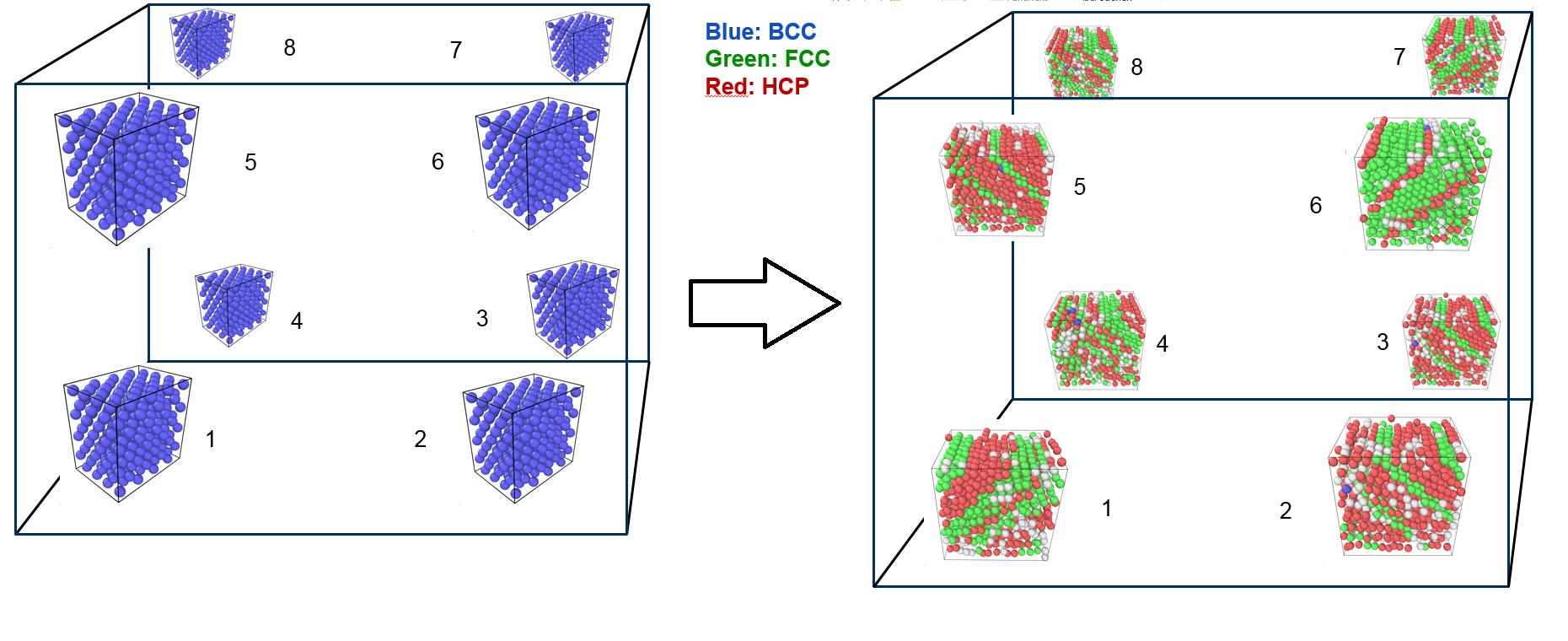# Multiscale simulation of phase change

Completed Research project

Coupling of continuum with molecular dynamics for the simulation of multiscale problems.

## Overview

• Atomistic-continuum coupling
• Nonlinear finite elementes
• Molecular dynamics
• FE²
• Phase transformation

## Project description

The goal of this research project ist the simulation of multiscale problems. These are problems, where the behavior on the nano scale influences the behavior on the micro scale. The approach to solve this problem consists of the coupling of continuum with molecular dynamics (MD). The continuum is represented via the finite element mehthod (FE). Since the last few decades a lot of research has been going on to find an appropriate coupling scheme and multiple methods have been developed. Those methods are either energy-based (e.g. the quasi continuum method) or force-based (e.g. the AtC-method). In this research project a different approach shall be taken similar to the FE²-method. Molecular sub problems shall be solved at the Gaussian points. The method is performed dynamically, both on the FE level and the MD level. The advantage of dynamic simulation is that the temperatur is integrated into the model explicitly. Both thermal strain and the strain-stress curve depend on the temperature. In addition is might also be possible to include grid changes, e.g. Iron changes from a centered cubic to face-centered cubic at high temperature.

### Molecular dynamics

The sub problem at each Gauß point is a small molecular dynamics box with periodic boundary conditions. From the finite element level the deformation gradient is calculated and used to deform the molecular dynamics box. On the MD level the virial stress (and potentially the elasticity tensor) are calculated and transferred to the FE level. With this information the stiffness matrix or the vector of the internal node forces can be calculated.

### Nonlinear finite elements

The finite element simulation part is nonlinear and dynamic. The stress measure is the second Piola-Kirchhoff stress and the strain measure the Green-Lagrange strain. Dynamic simulations do not require the stiffness matrix and the internal node forces are sufficient. This means that on the MD level only the current stress needs to be computed (e.g. as virial stress). The virial stress is equivalent to the Cauchy stress.

### Phase transformation

Pure Iron (ferrite or alpha-iron) changes phase at 1184 Kelvin from a body-centered structure to a face-centered structure (also called austenite or gamma-iron).  This transformation can also be simulated with the proposed method.Phase change within the sub problems of the hexahedron from body-centered cubic (BCC, blue) to face-centered cubic (FCC, green).

## Project data

Project titel:
Model-adaptive Atomistic-continuum Coupling for Multiscale Simulation of Metals
Funding:

DFG/ Cluster of Excellence in Simulation Technology (EXC 310/2)
Researcher:
Tobias Willerding